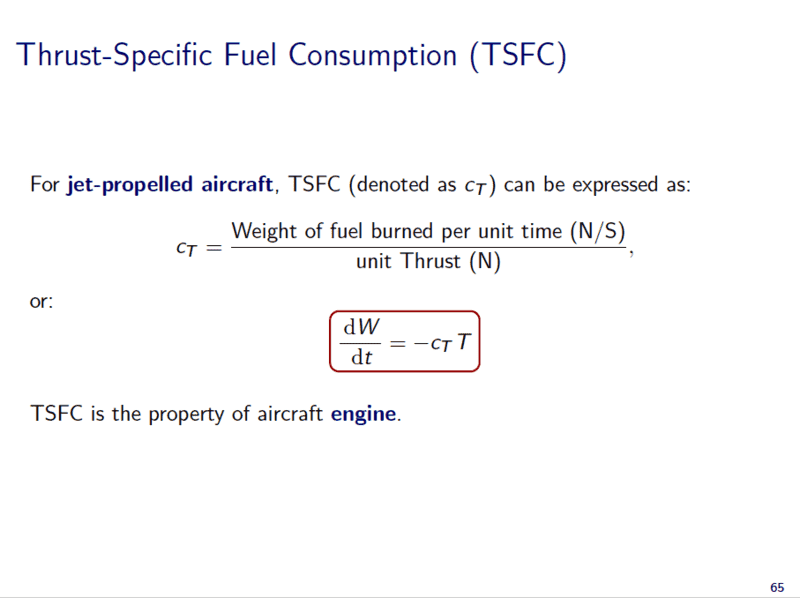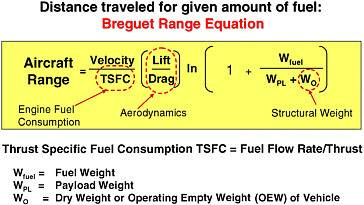# Unit conversion:lbm/lbf*h to (N/s)/N

Gold Member

## Homework Statement

Given Thrust-specific fuel consumption cT=0.5279lb/lbf*h... as other units in the question are in SI unit, I have to convert it to (N/s)/N first...

## Homework Equations

1 lbf = 4.448 N
1 lbm = 0.4536 kg
Thrust-specific fuel consumption = weight of fuel burned per unit time (N/s) per unit thrust (N)

## The Attempt at a Solution

cT=0.5279lb/lbf*h= (0.5279lb/lbf*h)* (4.448 N/lbf) / (0.4536 kg/lbm) / (3600s/h) = 1.49e-5 kgN-1s-1
which the unit of my answer stands for mass per force per time (kg/Ns) instead of force per time per force(N/Ns)...
where in the unit conversion have i done wrong??? thank you very much!

mfb
Mentor
Oh the joy of exotic unit systems. Not only the SI part doesn’t match, the imperial units don’t cancel each other either: As an example, you have lbf in the denominator twice and nothing to cancel it with. You’ll have to reconsider what to multiply and what to divide.

Gold Member
I have also tried to put 1lbf=1lbm*g=32.2 lbm^2 ft^-2,
then, the whole unit become lbm ft^2 h^-1
which also do nothing in manipulating the units at the end...

to be honest, this is my first course which i have to deal with imperial units, so i am not sure if my work is correct...

jbriggs444
Homework Helper

## The Attempt at a Solution

cT=0.5279lb/lbf*h= (0.5279lb/lbf*h)* (4.448 N/lbf) / (0.4536 kg/lbm) / (3600s/h) = 1.49e-5 kgN-1s-1
It might be easier to read and understand if you multiplied by every conversion factor instead of multiplying by some and dividing by others.

Typesetting that can be a pain. But maybe you could use [CODE] and [/CODE] tags to improve the presentation like this.

[CODE]
Code:
0.5279 lb   4.448 N     1 lbm
--------- * ------- * --------- * ...
1 lbf h     1 lbf    0.4536 kg
[/CODE]

Or you could get fancy and use LaTex. Key in

$\$\frac{0.529\ lb}{1\ lbf\ h} \cdot \frac{4.448\ N}{1\ lbf} \cdot \frac{1\ lbm}{0.4536\ kg} \cdot\ ...$\$​

and get
$$\frac{0.529\ lb}{1\ lbf\ h} \cdot \frac{4.448\ N}{1\ lbf} \cdot \frac{1\ lbm}{0.4536\ kg} \cdot\ ...$$

Last edited:
•scottdave
Ray Vickson
Homework Helper
Dearly Missed
It might be easier to read and understand if you multiplied by every conversion factor instead of multiplying by some and dividing by others.

Typesetting that can be a pain. But maybe you could use [CODE] and [/CODE] tags to improve the presentation like this.

[CODE]
Code:
0.5279 lb   4.448 N     1 lbm
--------- * ------- * --------- * ...
1 lbf h     1 lbf    0.4536 kg
[/CODE]

Or you could get fancy and use LaTex. Key in

$\$\frac{0.529\ lb}{1\ lbf\ h} \cdot \frac{4.448\ N}{1\ lbf} \cdot \frac{1\ lbm}{0.4536\ kg} \cdot\ ...$\$​

and get
$$\frac{0.529\ lb}{1\ lbf\ h} \cdot \frac{4.448\ N}{1\ lbf} \cdot \frac{1\ lbm}{0.4536\ kg} \cdot\ ...$$

The "CODE" version actually looks better than the LaTeX version if we don't take care to use the "\text" command to convert math font to text font. When we do that we get
$$\frac{0.529\,\text{lb}}{1\, \text{lbf h}} \cdot \frac{4.448\, \text{N}}{1\, \text{lbf}} \cdot \frac{1\, \text{lbm}}{0.4536\, \text{kg}} \cdots$$

•jbriggs444
jbriggs444
Homework Helper
The "CODE" version actually looks better than the LaTeX version if we don't take care to use the "\text" command
Thank you for that tip!

Gold Member
regardless the way I typed the equation, is there ways so that I can obtain the unit as (N/S)/N? (I am not able to corrected the original post...)
Thank you!

Gold Member
let me rewrite the formula here:
$$\ cT=\frac{0.5279lb}{lbf\cdot h}=\frac{(0.5279lb}{lbf\cdot h})\cdot(\frac{4.448N}{lbf})\cdot(\frac{0.4536kg}{lbm})^{-1}\cdot\left(\frac{3600s}{h}\right)^{-1}=1.49e-5\ kgN^{-1}s^{-1}$$

mfb
Mentor
You still have lbf in the denominator twice. But it looks like the original value has lbf in the nominator as well, so this conversion is much easier than you think.

Gold Member
or is lb=lbf?
$$\ cT=\frac{0.5279lb}{lbf\cdot h}=\left(\frac{0.5279lbf}{lbf\cdot h}\right)\cdot(\frac{4.448N}{lbf})\cdot(\frac{0.4536kg}{/lbm})^{-1}\cdot\left(\frac{3600s}{h}\right)^{-1}=1.49e-5\ kgN^{-1}s^{-1}$$

Gold Member
or like this??
$$\ cT=\frac{0.5279lb}{lbf\cdot h}=\left(\frac{0.5279lbf}{lbf\cdot h}\right)\cdot\left(\frac{3600s}{h}\right)^{-1}=1.467e-4\ s^{-1}$$

mfb
Mentor
Looks like it. N/N=1 so that can be included easily.

Gold Member
0.5279lb
but when I searched online,the numerator lb is lbm instead of lbf... so it cant be cancel out that easily
or else lb=lbf or kg doesn't really matter in the unit, and there is only unit of time^-1 there

Nidum
Gold Member
Specific fuel consump:(jet a/c) lb/hr. lb thrust = 0.283 x 10-4 kg/Ns

That's the aircraft industry standard conversion . Note the use of Kg and not N in top line on RHS .

•mfb
Nidum
Gold Member
In any conversion with mixed or odd units always check for consistency of dimensions on both sides of the conversion .

Gold Member
Specific fuel consump:(jet a/c) lb/hr. lb thrust = 0.283 x 10-4 kg/Ns
cT=0.5279lb/lbf*h= (0.5279lb/lbf*h)* (4.448 N/lbf) / (0.4536 kg/lbm) / (3600s/h) = 1.49e-5 kgN-1s-1
That should means my numeral calculation is correct :-)
That's the aircraft industry standard conversion . Note the use of Kg and not N in top line on RHS .This is the relevant lecture slide of my course... Do you think what mentioned by that is wrong? or they are somehow equivalent but by interpreting in different way? Thank you very much!

mfb
Mentor
It is the same quantity but multiplied by g, the gravitational acceleration. Why? Who knows. For rockets this is very common (Isp in seconds).

Gold Member
It is the same quantity but multiplied by g, the gravitational acceleration
multiply by g? then it has difference of 9.81!
when i use Breguet Range Equation (see the picture below), should i use cT with unit of N/Ns or kg/Ns?thanks.

Nidum
Gold Member
Lift/drag and the log term are both dimensionless .

Range = a dimensionless constant x Velocity/TSFC

Dimensionally : L = L T-1 / Dimensions of TSFC

(Edit) or dimensions of TSFC = T-1

Incidentally fuel 'weight' is sometimes expressed in Kg rather than N because it makes the unit independent of g . This is important in some flight dynamics calculations because g force as experienced by the fuel in a flight vehicle can vary considerably .

Last edited:
mfb
Mentor
multiply by g? then it has difference of 9.81!
No, the difference is 9.81 m/s2.
when i use Breguet Range Equation (see the picture below), should i use cT with unit of N/Ns or kg/Ns?
View attachment 213073
thanks.
That needs N/(Ns).

Gold Member
Incidentally fuel 'weight' is sometimes expressed in Kg rather than N because it makes the unit independent of g . This is important in some flight dynamics calculations because g force as experienced by the fuel in a flight vehicle can vary considerably .
So I have to multiply g in order to calculate range, right? Let me type my unit conversion here again:
$$\ cT=\frac{0.5279lb}{lbf\cdot h}=\frac{(0.5279lb}{lbf\cdot h})\cdot(\frac{4.448N}{lbf})\cdot(\frac{0.4536kg}{lbm})^{-1}\cdot\left(\frac{3600s}{h}\right)^{-1}$$
$$\ =1.49e-5\ kgN^{-1}s^{-1}\ \cdot\frac{9.81m}{s^2}=1.46e-4\ \frac{N}{Ns}$$
Is it correct and be ready to substitute it into Breguet Range Equation? Thank you so much!

jbriggs444
Homework Helper
So I have to multiply g in order to calculate range, right? Let me type my unit conversion here again:
$$\frac{0.5279 \text{ lb}} {\text{lbf} \cdot \text{h}} \cdot \frac{4.448 \text{ N}}{\text{lbf}}$$
[TeX formula cleaned up and trimmed down]

As has been pointed out repeatedly, this is the wrong way to proceed. You have lbf in the denominator and are multiplying it by a term with lbf in the denominator.

Also, your habit of taking the conversion terms and applying a (-1) exponent seems silly. Just turn the fraction upside down instead, putting the numerator on the bottom and the denominator on the top. That makes it much easier to read and keep track of everything.

Chestermiller
Mentor
As a guy who has been working with these unit all my life, I can tell you that 1 lbm/hr is the same as 0.4536 kg/hr. The weight of this is (0.4546)(9.8)=4.448 N/hr. And we know that 1 lbf=4.448 N. So, to convert from imperial to metric in this case, all you need to do is divide by 3600.

Gold Member
$$\ cT=\frac{0.5279lb}{lbf\cdot h}=\frac{(0.5279lb}{lbf\cdot h})\cdot\left(\frac{3600s}{h}\right)^{-1}=1.46e−4 N/Ns$$​